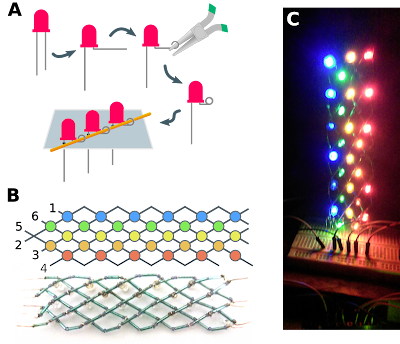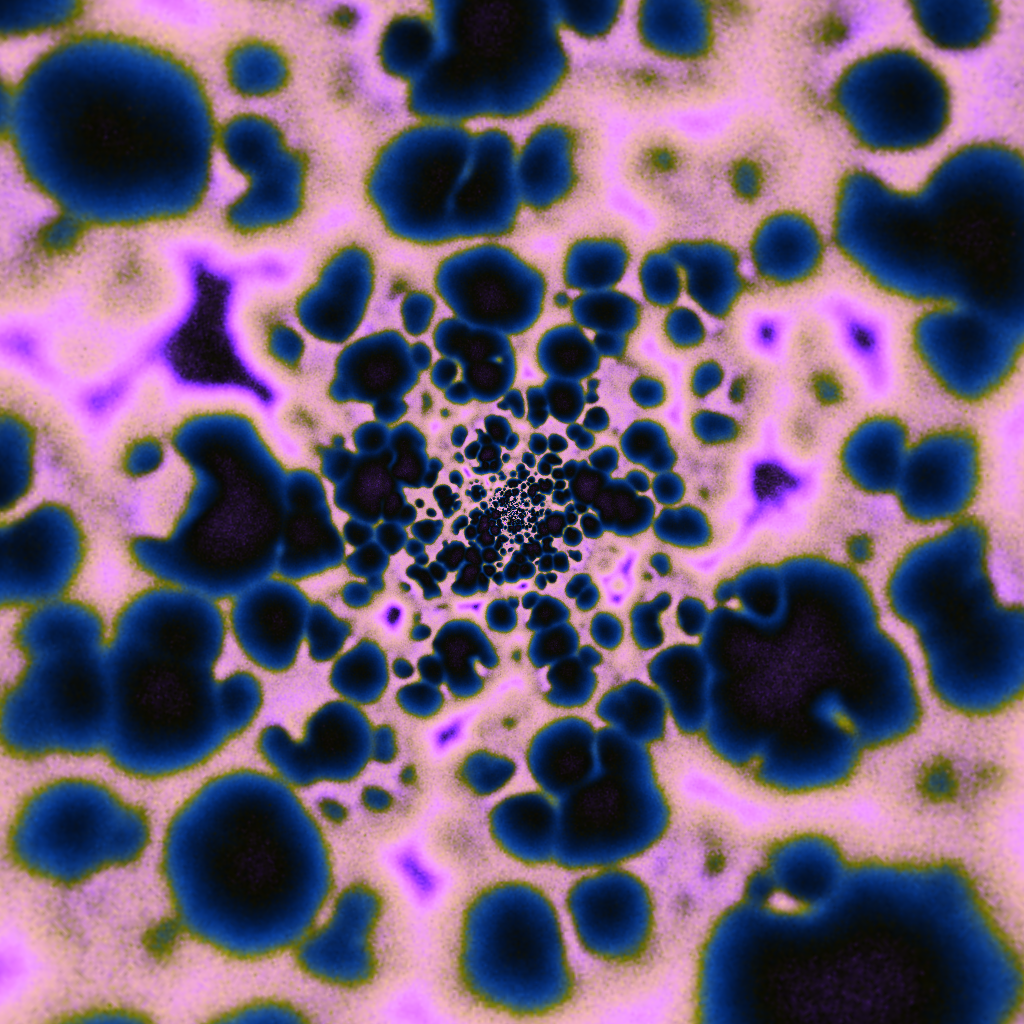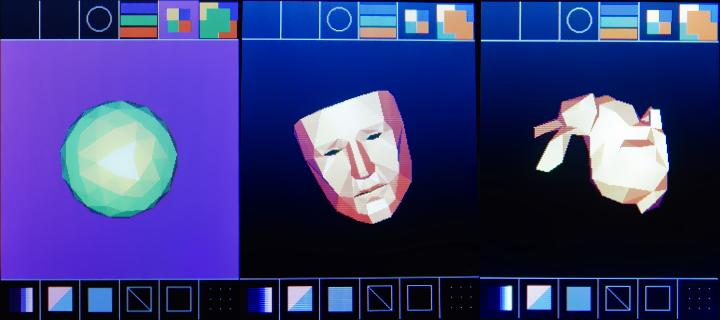## 20190207

Have you ever wanted to build a LED matrix display using hand-crafting methods, such as sewing, weaving, or papercraft? Designing and fabricating complex LED projects by hand, while making use of limited input-output (i/o) pins on microcontrollers/Arduino, is challenging. Previous posts have covered how to organize LED matrix displays using "Charlieplexing", which simplifies printed circuit board (PCB) assembly and makes efficient use of i/o pins. But, what if we don't want to go through the hassle of ordering a custom PCB, or what if we want to work with individual LEDs in a craft project? Cost is also a factor: a cardstock "papercraft" display could be built from a cheap Arduino-compatible board and bulk LEDs for under $5 on Ebay, far cheaper than even the cheapest LED scrolling marquees. For a brief review, LED multiplexing involves attaching multiple LEDs sharing a common anode (+ side) or cathode (- side) arrangement. Only one row or column is active at a time, and rows/columns are scanned rapidly so that all lights may appear to be on. Carlieplexing is similar, but uses the same set of microcontroller pins for the anodes and the cathodes. Grid locations where the anode and cathode are now the same pin are excluded (blacked out LEDs, right, below). A previous post covered how to make the best use of the layout space for Charlieplexing LED matrices. For hand-crafted projects, we'd like a way to build multiplexed layouts as simply as possible, without extra "wires" tracing throughout the project. This can be done by using a diagonal slice of the Charlieplexing grid layout, which allows the driving lines to wrap around the edges of the project: This diagonal layout is unconventional, and at first might not seem to lend itself to projects that need a rectangular grid, for example to display text. The geometry is flexible, however, and allows for distortion and rearrangement of the LED positions. For example, individual rows can be shifted to create a rectangular layout (a), and the grid may be stretched to create a hexagonal grid (b), which is convenient for tiling red, green, and blue LEDs for a full-color display (but see caveats about mixing different color LEDs in the example project, below). Since the layout is periodic in the long axis, it can be wrapped onto polar (c) or cylindrical coordinates (d), creating some fun possibilities! ## Test project: LED bead weavingTo illustrate this layout approach, I've built a small woven display using a "bead weaving" approach. Individual LEDs are strung on a copper wire, which is decorated with glass beads for insulation and stiffness. For this design, I first determined the layout geometry on paper, and mounted the LEDs in cardboard for stability when soldering, clipping the cardboard away once the project was complete. It's hard to replace damaged LEDs, so take care when assembling and testing the project. If mixing different color LEDs, ensure that the lowest forward voltage is no less than half the highest forward voltage, otherwise the current might "skip" higher-voltage LEDs by passing through two low-voltage ones. Check the maximum instantaneous current ratings for your LEDs, and be sure to add current-limiting resistors. I made several mistakes in this assembly, including forgetting to leave gaps in the beads to allow for soldering, and soldering at a temperature high enough to damage the LEDs. Be careful not to overheat the LEDs: without the added mechanical stability of a PCB, melting or softening of the plastic can damage the chip inside. To construct:(A) Design the layout grid on stiff cardboard. Use round-nose pliers to form the LED leads into loops, (just the cathodes at first). Place the LEDs in the cardboard layout, then form the loops for the anodes other side. Thread copper wire through the project to connect the LEDs, optionally attaching glass beads for insulation and aesthetics, and solder. (B) This example project uses 6 control lines to drive 5 columns of colored LEDs. (C) Finished project; (some LEDs were damaged in assembly). The Arduino source code scans each light one-at-a-time. ## 20170126 ### Dissociation between sustained single-neuron spiking β-rhythmicity and transient β-LFP oscillations in primate motor cortex Some of my thesis work has just been published! Rule et al. 2017 explores the neurophysiology of beta (β) oscillations in primates, especially how single-neuron activity relates to population activity reflected in local field potentials (a.k.a. "brain waves"). β (~20 Hz) oscillations occur in frontal cortex. We've known about them for about a century, but still don't understand how they work or what they do. β-wave activity is related to "holding steady", so to speak. β is dysregulated in Parkinson's, in which movements are slowed or stopped. β is also reduced relative to slow-wave activity (θ) in ADHD, a disorder associated with motor restlessness and hyperactivity. I looked at β oscillations during movement preparation, where β seems to play a role in stabilizing a planned movement. We found that single neurons had very little relationship to the β-LFP brain waves. However! This appears to be for a good reason: the firing frequencies of neurons store information about the upcoming movement, and so are diverse and cannot lock to a single frequency. Anyone who's played in an orchestra knows that when notes are just slightly out of tune, you get interference patterns called beats. The same thing is happening in the brain, where many neurons firing at slightly different "pitches" cause β-LFP fluctuations, even though the underlying neural activity is constant. This result provides a new explanation for how β-waves can appear as "transients" during motor steady-state: the fluctuations are cased by "beating", rather than changes in the β activity in the individual neurons. This differs from the prevailing theory for the origin of β transients in more posterior brain regions. ### Optogenetic Stimulation Shifts the Excitability of Cerebral Cortex from Type I to Type II: Oscillation Onset and Wave Propagation A new paper by Stewart Heitmann et al. could help us understand what happens when we stimulate cerebral cortex in primates using optogenetics. Modeling how the brain responds to stimulation is important for learning how to use this new technology to control neural activity. Optogenetic stimulation elicits gamma (~50 Hz) oscillations, the amplitude of which grows with the intensity of light stimulation. However, traveling waves away from the stimulation site also emerge. It's difficult to reconcile oscillatory and traveling-wave dynamics in neural field models, but Heitmann et al. arrive at a surprising and testable prediction: the observed effects can be explained by paradoxical recruitment of inhibition at low levels of stimulation, which changes cortex from a wave-propagating medium to an oscillator. (Excitation later overwhelms inhibition, giving rise to the observed gamma oscillations.) ## 20160719 ### Wilson-Cowan neural fields in WebGLWebGL offers a simple way to write portable general-purpose GPU (GPGPU) code with visualization. The Github repository WebGPGPU walks through a series of examples to bring up a port of Wilson-Cowan neural field equations in WebGL. The Wilson-Cowan equations are a mean-field approximation of neural activity under the assumption that spiking is asynchronous, and that observations are averaged over a large number of uncorrelated neurons. Wilson-Cowan neural fields have been used to describe visual hallucinations, flicker phosphines, and many other wave phenomena in the brain. The Wilson-Cowan simulation demo is rudimentary at the moment, but contains a couple presets and rich and diverse dynamics are possibly by varying parameters. For entertainment, there is also an example of a full-screen pattern forming system mapped to (approximate) retinal coordinates using the log-polar transform. Other cool WebGL implementations pattern-forming systems include the Gray-Scott reaction diffusion equations by pnmeila and Felix Woitzel's reaction diffusion and fluid dynamics simulations. Robin Houston's reaction diffusion implementation is also a good reference example for learning WebGL coding, and Robter Muth's smoothlife implementation is mesmerizing. The hope is that the examples in the WebGPGPU project provide a walk-through for how to build similar simulations in WebGL, something that is not immediately obvious if following existing WebGL tutorials. ### Technical notes I've avoided using extensions like floating point textures that aren't universally supported. Hopefully the examples in WebGPGPU will run on most systems, but compatibility issues likely remain. Most of the examples store floating point values in 8-bit integers. This works for the firing rate version of the Wilson-Cowan equations because the firing rate is bounded between 0 and 1, so fixed precision is adequate. There are some caveats here. For example, how floating point operations are implemented, and how floats are rounded when being stored in 8-bit values, are implementation dependent. These simulations a quite sensitive to parameters and rounding errors, so different implementations can lead to different emergent dynamics. A partial workaround is to manually control how floats are quantized into 8-bit values. Floats can also be stored in 16 or 32 bit fixed-point precision, at the cost of performance. My understanding is that the default precision for fast floating point operations on the GPU is fairly low, such that 16 bits can capture all of the available precision for the [0,1] range. The quantization of state variables can lead to numerical errors if the integration step size is too small relative to the timescales of the system. In short, if states are changing too slowly, their increments becomes smaller than 1/256, and numerical accuracy degrades because state changes are rounded-out. Moving to 16-bit precision lessens this issue, but at a cost of an approximately 2-fold slowdown, and so is not implemented except in one example. Working with the WebGL library feels quite clumsy, because in essence the API exposes the GPU as a state machine. WebGPGPU includes some libraries that hide a lot of the boilerplate and expose a more functional interface for GPU shaders (kernels). For simulations on a grid, there is additional boilerplate to gain access to single pixes. One must tile the viewport with polygons, provide a default fragment shader, and set the viewport and canvas sizes to match the on-screen size. WebGPGPU hides this difficulty, but also walks through this process in the early examples to make it clear what is really happening. Passing parameters to shaders involves a fair amount of overhead in Javascript, which becomes prohibitive for shaders with a large number of parameters. However, compiling shaders has relatively little Javascript overhead. In practice it is faster to include scalar parameters as #defines rather than pass them as uniforms. WebGPGPU contains some routines to phrase shader compilation as a sort of 'partial evaluation'. This is a work in progress. ## 20160708 ### Numerically stable Viterbi algorithm in Python for hidden markov model state inference The Python demonstration of the Viterbi algorithm on Wikipedia is numerically unstable for moderate to large problem sizes. This implementation is phrased in terms of log probabilities, and so has better numerical properties. Please reuse, creative commons. def hmm_viterbi(Y,logP,logA,logB): ''' See https://en.wikipedia.org/wiki/Viterbi_algorithm Parameters ---------- Y : 1D array Observations (integer states) logP : array shape = (nStates ,) 1D array of priors for initial state given in log probability logA : array (nStates,nStates) State transition matrix given in log probability logB : ndarray K x N conditional probability matrix log probabilty of each observation given each state ''' K = len(logP) # Number of states T = len(Y) # Number of observations N = np.shape(logB) # Number of states Y = np.int32(Y) assert np.shape(logA)==(K,K) assert np.shape(logB)==(K,N) # The initial guess for the first state is initialized as the # probability of observing the first observation given said # state, multiplied by the prior for that state. logT1 = np.zeros((K,T),'float') # Store probability of most likely path logT1[:,0] = logP + logB[:,Y] # Store estimated most likely path T2 = np.zeros((K,T),'float') # iterate over all observations from left to right for i in range(1,T): # iterate over states 1..K (or 0..K-1 with zero-indexing) for s in range(K): # The likelihood of a new state is the likelihood of # transitioning from either of the previous states. # We incorporate a multiplication by the prior here log_filtered_likelihood = logT1[:,i-1] + logA[:,s] + logB[s,Y[i]] best = np.argmax(log_filtered_likelihood) logT1[s,i] = log_filtered_likelihood[best] # We save which state was the most likely T2[s,i] = best # At the end, choose the most likely state, then # Iterate backwards over the data and fill in the state estimate X = np.zeros((T,) ,'int' ) # Store our inferred hidden states X[-1] = np.argmax(logT1[:,-1]) for i in range(1,T)[::-1]: X[i-1] = T2[X[i],i] return X  ## 20151230 ### Better 3D graphics on the Arduino: avoiding flickering and tearing A while ago I purchased a cheap$4 Chinese LCD Arduino shield from Ebay (e.g 1 2 3). The board arrived with no documentation. Disassembling the shield revealed no ICs, indicating that the driver is integrated with the LCD itself. Upon request, the vendor provided an archive containing a few amusingly translated datasheets, as well as a copy of Adafruit's Arduino LCD drivers. Evidently the product is a clone of the Adafruit LCD shields, and uses the ILI9341 LCD driver. I had hoped to use the display to show short animated GIF loops. This is not, in practice, possible. Test animations loaded slowly, with noticeable flicker and vertical tearing. The Arduino does not have enough speed or bandwidth to render full-screen animation frames, but what about 3D vector graphics?## 20150827

### Wilson-Cowan neural field model, in HTML/JavaScript, in under 512 bytesThis started essentially as a bet made at a bar. It turns out that one can make a Wilson-Cowan neural field demo in under 512 bytes in HTML/JavaScript, so long as one is willing to use invalid HTML that will only render in Firefox.

View demo hosted on Dropbox (Firefox only)

For these parameters, the simulation starts out with noise. Radiating waves slowly form. Eventually the solution converges to spiral waves.

Here's the source. There are 466 bytes of actual JavaScript and and 491 bytes total.
<canvas><script>c=document.body.lastChild
c.width=c.height=W=256
Z=c.getContext('2d')
l=Z.getImageData(0,0,W,W)
N=W*W
I=()=>Float32Array(N)
t=I();b=I();f=I();g=I();h=I()
K=(w,t,W)=>{for(i=N;i--;){w[i]=0;for(j=3;j--;)w[i]+=(j*4+2&7)/5*t[W*j-W+i&N-1]}}
w=()=>{K(t,b,1);K(g,t,W);K(t,f,1);K(h,t,W)
for(i=N;i--;){B=(j)=>.7/(1+Math.exp(j+3+4*h[i]-5*g[i]-Math.random()))
b[i]=B(6*h[i]-g[i])+b[i]*.3
f[i]=B(1)*.13+f[i]*.91
l.data[i*4+3]=b[i]*(W-1)}Z.putImageData(l,0,0);setTimeout(w,0)};w()</script>


In addition to refactoring expressions to reduce size and using invalid HTML, the code also takes advantage of the facts that
• indecies into a 256 x 256 array can be wrapped horizontally and veritcally by masking with 0xffff, yielding periodic boundary conditions for free
• a succinct way to get a grayscale canvas on a white page is to just write to the alpha bytes of the canva's image data
• "for(i=N;i--)" iterates over 0 to N-1 in reverse order, and is shorter than "for(i=0;i<N;i++)"
c=document.body.lastChild// get the canvas (it's the only element)
c.width=c.height=W=256   // set canvas size (and width constant)
Z=c.getContext('2d')     // get graphics context
l=Z.getImageData(0,0,W,W)// get handle to image data buffer
N=W*W                    // define a constant the length of the data buffer
I=()=>Float32Array(N)    // define short function for initializing buffers
t=I()                    // make scratch for convolution intermediate values
b=I()                    // this will be the E-cell buffer
f=I()                    // this will be the I-cell buffer
g=I()                    // buffer to hold blurred E-cell state
h=I()                    // buffer to hold blurred I-cell state
K=(w,t,W)=>{             // function for separable convolution.
// blurs from NxN buffer t into 256x256 buffer w
// W is the step size. If it is 1, the blur will be
// horizontal. If it is 256, the blur will be
// vertical. Gaussian is convolution is separable
// in x/y, so we can do the 2D blur by first
// doing the x and then the y
for(i=N;i--;){       // iterate over all points
w[i]=0;          // initialize accumulator to zero
for(j=3;j--;)    // iterate over kernel
// The expression (j*4+2&7) saves one character over
// we wrap indecies in x and y by masking with
// 0xffff i.e. N-1
w[i]+=(j*4+2&7)/5*t[W*j-W+i&N-1]
}
}
w=()=>{
K(t,b,1)             // convolve E-cell buffer in x direction
K(g,t,W)             // convolve E-cell buffer in y direction
K(t,f,1)             // convolve I-cell buffer in x direction
K(h,t,W)             // convolve I-cell buffer in y direction
for(i=N;i--;){       // for every point
// B is an update function
// includes firing rate nonlinarity and some of the
// Wilson-cowan update -- expression have been
// refactored to minimize size
B=(j)=>.7/(1+Math.exp(j+3+4*h[i]-5*g[i]-Math.random()))
b[i]=B(6*h[i]-g[i])+b[i]*.3 // update E-cell field
f[i]=B(1)*.13+f[i]*.91      // update I-cell field
l.data[i*4+3]=b[i]*(W-1)    // store E-cell value in alpha channel
}
Z.putImageData(l,0,0);          // update image
setTimeout(w,0)                 // start next frame
};
w()                                 // start simulation